Please review your test results by moving cursor to answer sheet icon.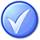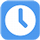1.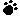Pareto's Law states that eighty percent of the ______ are due to twenty percent of the _______. This is also known as the 80/20 rule.

A) problems/causes
B) causes/problems
C) work/effort
D) effort/work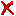Your answer=B, Correct answer=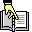, Work Sheet:

2.A)
B)
C)
D)

Your answer=, Correct answer=,

3.A)
B)
C)
D)

Your answer=, Correct answer=,

4.A)
B)
C)
D)

Your answer=, Correct answer=,

5.A)
B)
C)
D)

Your answer=, Correct answer=,